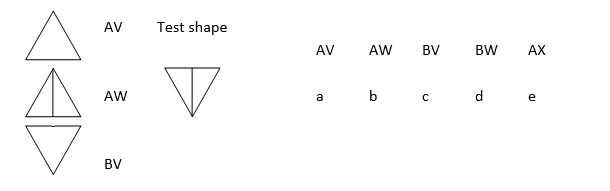# NVR - Looking at a Shape and Making a Code (1)

Example – Decide on the meaning of the code on the left. Each letter means something and can be determined by looking at the symbols. Then look at the test shape and decide which of the given code letters is an accurate description of it.This is, in my experience, a relatively straightforward section of the test and it does not always appear on non-verbal papers. However, there are some tricky elements and lots of things to watch out for.

Let’s work out how to solve these sorts of questions. The first step is to find out what is in common between the elements of the initial shapes which share code letters. Let’s look at the options:

The first two shapes are both coded ‘A’

The first and third shape are both coded ‘V’

We can find out what the code letter ‘A’ means by seeing what is in common with the first two shapes and is not shared with the other. The answer, of course, is that the first two shapes are triangles pointing upwards while the bottom one faces downwards. This means that ‘A’ and ‘B’ refer to the direction the triangle points, so our test shape, as it points downwards, must be coded ‘B’.

‘V’ is used to show something which the first and third shape have in common and not the second, so seems to relate to being empty or having a line through the middle. Shapes coded ‘V’ have nothing in the middle while a shape with the code ‘W’ has a line down the middle. As the test shape has a line through it, it must be coded ‘W’ and the overall code is therefore ‘BW’ (answer ‘d’).

Technique tip:

The way to answer these questions is to find the similarities between objects that share a code letter and work from there. In order to do this you need to think along the lines of question setters, so bear in mind the list of things which I provided at the start of this section. Shape, size, shading, lines, number, and rotation are key elements in this section.

Beware of the ‘repeat’ letter combinations. For example, in the question above the first three possible answers are all codes which have been used to describe other shapes. It is very rare to see a replication of answers for different shapes. Discourage children from putting an answer which is the same code as one of the earlier shapes unless they are quite definite.

Go to the next Non Verbal Reasoning working example - Looking at a Shape and Making a Code (2)